# NCERT Solutions for Class 10 Maths Chapter 14 Statistics Ex 14.3

These NCERT Solutions for Class 10 Maths Chapter 14 Statistics Ex 14.3 Questions and Answers are prepared by our highly skilled subject experts.

## NCERT Solutions for Class 10 Maths Chapter 14 Statistics Exercise 14.3Question 1.
The following frequency distribution gives the monthly consumption of electricity of 68 consumers of a locality. Find the median, mean and mode of the data and compare them.Solution:
The cumulative frequency distribution of given frequency distribution given below.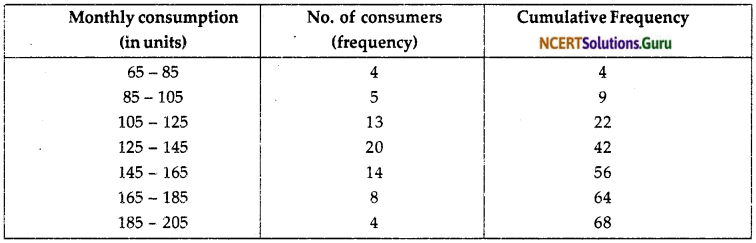(i) Median
$$\frac { n }{ 2 }$$ = $$\frac { 68 }{ 2 }$$ = 34
Therefore, median class is (125 -145)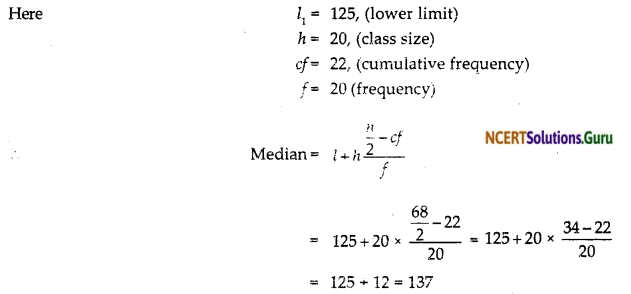∴ Median of the given frequency distribution is 137 units.

(ii) Mode Here f1 = 20, f2 = 14, f0 = 13, l = 125, h = 0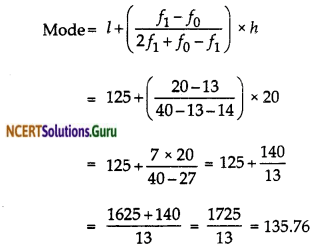∴ Mode of the given data is 135.76

(iii) Mean
We know that relation between mean, mode and median is
3 Median = Mode + 2 Mean
2 Mean = 3 Median – Mode
2 Mean = 3 (137) – (135.76)
= 411 – 135.76
= 275.24
Hence Mean = 137.62
∴ Mean of the given data is 137.62.

online median calculator tool makes the calculation faster, and it displays the measures of central tendencies in a fraction of seconds.Question 2.
If the median of the distribution given below is 28.5, find the values of x and y.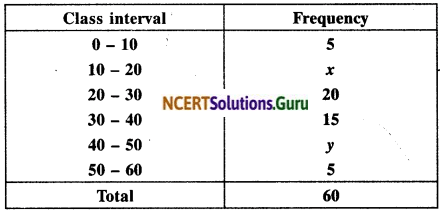Solution: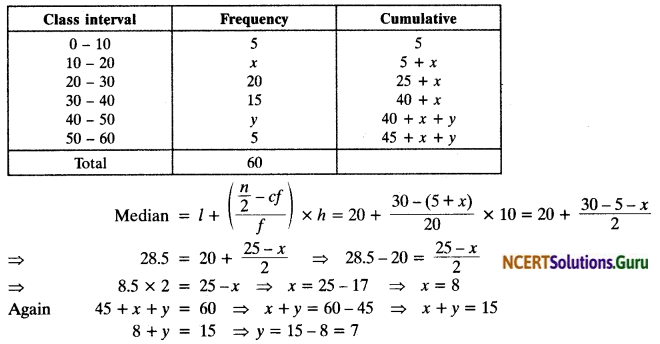Question 3.
A life insurance agent found the following data for distribution of ages of 100 policy holders. Calculate the median age, if policies are given only to persons having age 18 years onwards but less than 60 years.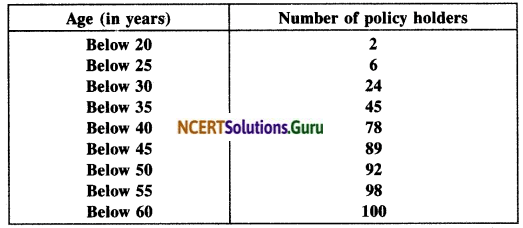Solution: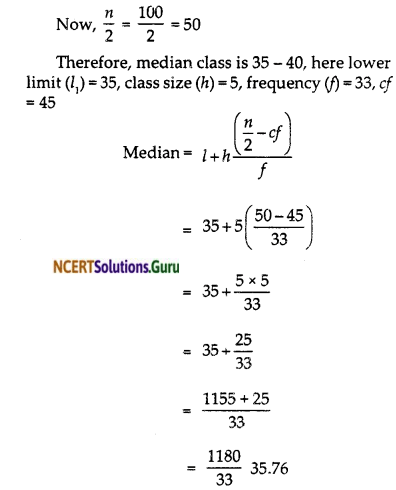Hence, median age is 35.76 years.

Use our Special Age Calculator to find out your upcoming special age in seconds, minutes, hours, days and weeks.Question 4.
The lengths of 40 leaves of a plant are measured correct to nearest millimetre, and the data obtained is represented in the following table:Find the median length of the leaves.
(Hint: The data needs to be converted to continuous classes for finding the median since the formula assumes continuous classes. The classes then change to 117.5 – 126.5,126.5 -135.5,… 171.5 -180.5.)
Solution: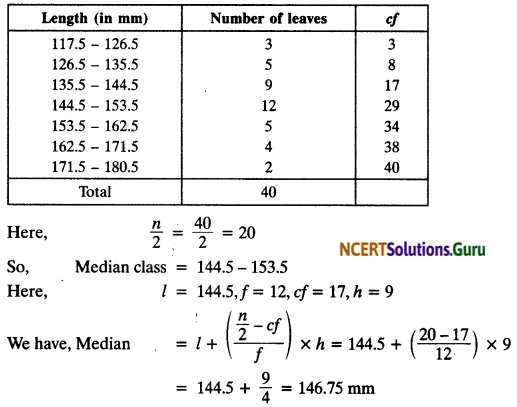Question 5.
The following table gives the distribution of the lifetime of 400 neon lamps:Find the median lifetime of a lamp.
Solution:Question 6.
100 surnames were randomly picked up from a local telephone directory and the frequency distribution of the number of letters in the English alphabet in the surnames was obtained as follows:Determine the median number of letters in the surnames. Find the mean number of letters in the surnames. Also, find the modal size of the surnames.
Solution: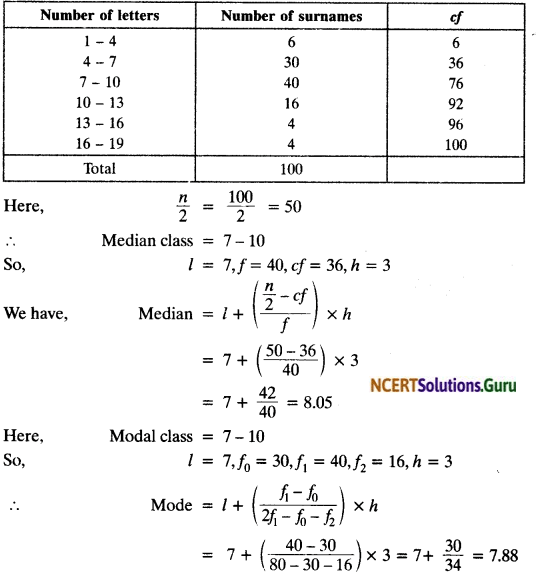For mean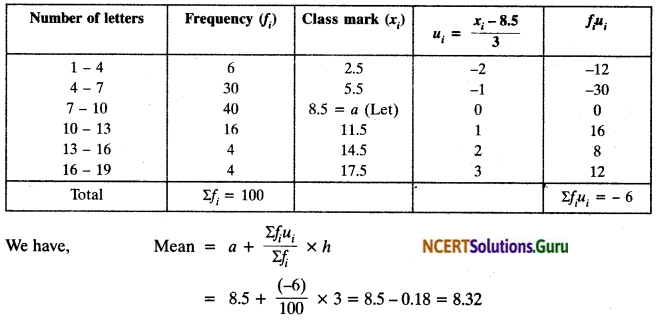Question 7.
The distribution below gives the weight of 30 students of a class. Find the median weight of the students.Solution: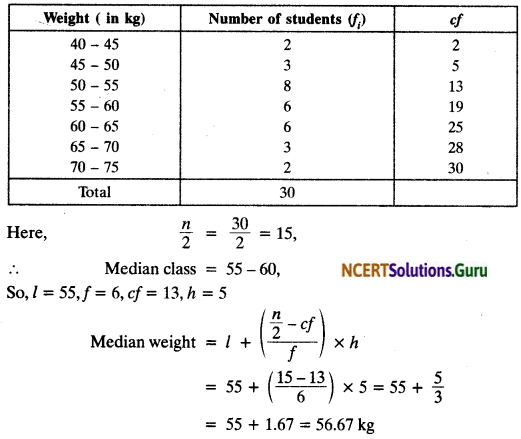error: Content is protected !!